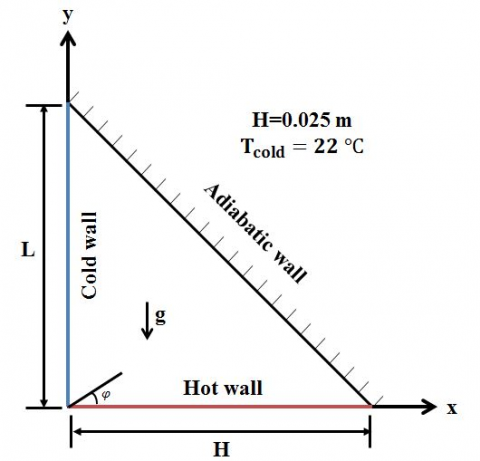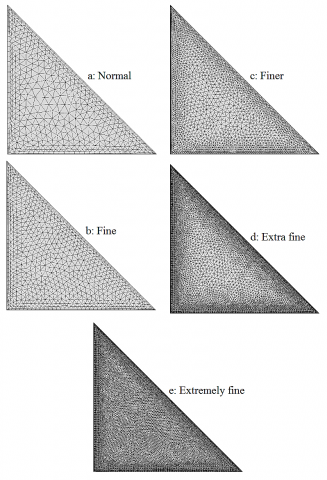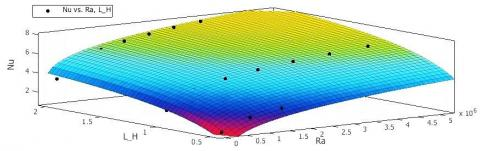# CFD Modeling of Natural Convection in Right-Angled Triangular Enclosures

CFD Modeling of Natural Convection in Right-Angled Triangular Enclosures

Department of Chemical and Petroleum Engineering, Sharif University of Technology, Azadi Avenue, Tehran, Iran 11365-8639

Corresponding Author Email:
smmirabedin@alum.sharif.edu
Page:
503-506
|
DOI:
https://doi.org/10.18280/ijht.340322
|
Accepted:
|
Published:
30 September 2016
| Citation

OPEN ACCESS

Abstract:

Two-dimensional numerical simulations have been performed to study natural convection in right-angled triangular enclosures filled with water considering different aspect ratios. Continuity, momentum and energy equations are solved assuming Boussinesq approximation utilizing COMSOL. Effect of Rayleigh number, Ra, on heat transfer rate is investigated by showing Nusselt number, Nu, for a range from 1×104 to 1×107. It is shown that increasing aspect ratio of the cavity increases averaged Nusselt number in a cavity heated from below. Finally, a correlation for heat transfer rate is developed considering the effect of aspect ratio using simulation results.

Keywords:

Natural convection, Numerical simulation, Nusselt number, Rayleigh number, Right-angled enclosure.

1. Introduction

Triangular cavities have a wide range of applications in solar collectors and electronic devices as well as heating and ventilation in buildings with inclined roofs. Many researchers have been studying natural convection phenomenon in enclosures. For instance, Lorenzini et al. studied the geometry of cavities bathed by a fluid intruded into a cylindrical solid body. Concerning various cavity area fractions and cavity numbers, they optimized the shape of the inserted cavities . Aydin and Yesiloz worked on the buoyancy induced flow and heat transfer in a water-filled cavity experimentally and numerically . They observed that for Rayleigh numbers less than $10^{3}$ the effect of Rayleigh number becomes insignificant. Ghasemi and Aminossadati studied natural convection in a triangular enclosure, heated from its vertical side, for water-CuO nanofluid . Studying the effects of different variables such as solid volume fraction and Rayleigh number, they reported an enhancement in heat transfer rate for upper location of heat source for Rayleigh numbers. Ridouane et al. numerically modeled laminar natural convection in a triangular cavity using air as the working fluid . In their case, vertical side was considered as hot wall while cooling was done from the hypotenuse. It was found that heat transfer rate decreases with a decrease in apex angle. Sun and Pop numerically investigated heat transfer in a triangular enclosure filled with porous medium . Considering the effects of different parameters, such as Rayleigh number and size and position of heater, they reported an enhancement in heat transfer with increasing solid volume fraction. Another study by Yesiloz and Aydin considering the effect of the inclination angle and Rayleigh number on Nusselt number in an inclined quadrantal enclosure showed that heat transfer rate changes dramatically by increasing inclination angle for Rayleigh numbers between $1 \times 10^{5}$ and $1 \times 10^{7}$ . Despite their comprehensive experimental and numerical study, they did not provide any correlation regarding the effect of inclination angle on Nusselt number. Furthermore, Yesiloz and Aydin developed a correlation for an isosceles right-angled triangular cavity heated from below and cooled from the side wall observing an increase in Nusselt number with an increase in Rayleigh number . Kaluri et al studied heat transfer in a rectangular enclosure insulated from base considering different boundary conditions and aspect ratios . They found the highest heat flux for top angle of 15o. Although they compared different configurations and reported expressions for Nusselt number for both cases of constant wall temperature and constant heat flux, their correlations might not be practical in many cases since they reported separate correlations for each Prandtl number and top angle relating Nusselt and Rayleigh numbers. In addition, Akinsete and Coleman introduced a correlation for a triangular cavity heated from hypotenuse and cooled from below by curve fitting of numerical data . They reported that for their arrangement, Nusselt number decreases by increasing the height to base ratio of the triangular enclosure while increases by rising Rayleigh number.

From the literature review above, it can be concluded that although plenty of work has been done on cavities considering different shapes, working fluids and boundary conditions, few of them reported practical correlations for Nusselt number considering the effect of aspect ratio and Rayleigh number. In the present work, natural convection in triangular enclosures is studied for three different aspect ratios in cavities heated from below and cooled from the height.

2. Computational Model

Computational model of the enclosure is shown in Figure 1. The model consists of an adiabatic inclined wall (hypotenuse), which does not allow heat to pass across it as well as side and base walls, kept at cold and hot temperatures, respectively.

## figure_1.pngFigure 1. Computational domain of the enclosure

Considering laminar flow and neglecting the effect of radiation, the governing equations can be written in Cartesian coordinates as Eqs (1) to (3).

Continuity:

$\rho \nabla \cdot u=0$(1)

Navier-Stokes:

$\rho(u . \nabla) u=\nabla \cdot\left[-p I+\mu\left(\nabla u+(\nabla u)^{\tau}\right)\right]$(2)

Energy:Boussinesq assumption $\left(\rho g \beta\left[T-\frac{1}{2}\left(T_{H}+T_{C}\right)\right]\right)$ is considered for flow in negative z-direction.

$\rho C_{p} u . \nabla T=\nabla \cdot(k \nabla T)$(3)

Horizontal wall of the enclosure is at various constant hot temperature while the vertical side is at constant cold temperature (22 oC) in accordance with experimental conditions. Hypotenuse is kept thermally insulated. The ratio of height to base ratio, defined as aspect ratio (L/H), varies between three values of 0.5, 1.0 and 2.0 which are commonly used in practical applications.

3. Numerical Analysis

COMSOL 4.4 has been used to model the geometries and post-processing. All steady-state computations have been performed on an Intel i7 having a quad processor with 8 GB RAM and 2.0 GHz processor speed. Total tolerance has been considered as $1 \times 10^{-6}$  in all cases. Thermo-physical properties of water are considered as pre-defined expressions given in .

The definition presented by Yesiloz and Aydin is used to calculate the average Nusselt number for the base side of the cavity (see Eq (4)) .

$N u=\left.\frac{\frac{\pi}{2}}{\left(T_{H}-T_{C}\right)} \frac{\partial T}{\partial \varphi}\right|_{\varphi=0}$(4)

where $\varphi$  is the angle from base side in polar coordinates as shown in Figure 1. This definition makes Nu number, calculated on hot wall, more meaningful by giving the critical Nu=1 for Rayleigh number less than $10^{3}$  for isoscales triangular encosure. Detailed information about this definition can be found in [2,6]. To convert the derivation of temperature in Cartesian coordinates to polar coordinates, Eq (5) is considered in accordance with chain rule where r is the distance between a point on the domain and the origin in polar coordinates.

$\frac{\partial T}{\partial \varphi}=-\frac{\partial T}{\partial x}(r \sin \varphi)+\frac{\partial T}{\partial y}(r \cos \varphi)$(5)

Volume-averaged Rayleigh number is shown in Eq (6) considering the effect of natural convection in the enclosure.

$R a=\frac{\rho^{2} C_{p} g \beta\left(T_{H}-T_{C}\right) H^{3}}{k \mu}$(6)

Grid dependency of the isosceles triangular cavity is shown in Table 1 for five different mesh sizes. These mesh sizes are shown in Figure 2 as well.

In comparison with experimental observations, the last two cases were found as the most suitable gird densities. Hence, case 4 was considered due to its less conservation time.

$u=\frac{\partial \psi}{\partial y}, \quad v=-\frac{\partial \psi}{\partial x}$(7)

Table 1. Mesh dependency for isocletes triangular, $\mathrm{Ra}=2.45 \times 10^{6}$

 CASE MESH SIZE NAME NU 1 1000 Normal 5.841 2 1500 Fine 5.776 3 4600 Finer 5.633 4 12500 Extra fine 5.586 5 35000 Extremely fine 5.586

Stream function is defined as Eq (7). Numerical results in terms of isotherms and streamlines are compared with published data by Yesiloz and Aydin  in Figures 3-4.

## figure_2.pngFigure 2. Comparing different grid sizes for the isoscales triangular enclosure heated form below

4. Results and Discussion

Numerical simulations of laminar natural convection in a triangular cavity have been conducted for different Rayleigh numbers considering different hot temperatures. Computational results have been validated with existing results for an isosceles triangular cavity filled with water (see Figure 5). As can be seen, Nusselt number increases by increasing Rayleigh number in a range of $1 \times 10^{4}$ to $1 \times 10^{7}$.

Considering two other aspect ratios, an augmentation in the averaged Nusselt number was observed by an increase in aspect ratio (see Figure 6). In order to develop a correlation that considers the effect of aspect ratio as well as Rayleigh number, an equation in form of Eq (8) is suggested. Constants of this equation were found using curve fitting toolbox in MATLAB 2012 (shown in Table 2). This expression was found in agreement with simulation data for all aspect ratios and Rayleigh numbers as well as heat transfer rates (R2=0.986). This expression for natural convection in the enclosures is shown in Figure 7 as a function of Rayleigh number and aspect ratio as a 3D plot.

## figure_3.pngFigure 3. Comparing experimental stramlines by Yesiloz and Aydin  (a) and present numerical streamlines (b) in an isoscles triangular cavity for $\mathrm{Ra}=10^{5}$

## figure_4.pngFigure 4. Comparing dimensionless isotherms in an isosceles triangular enscolsure for $\mathrm{Ra}=10^{5}$ , (a): Yesiloz and Aydin , (b): Numerical simulation

## figure_5.pngFigure 5. Comparing numerical simulation results with experimental data for an isosceles triangular cavity heated from below (L/H=1)

Table 2. Constants of the suggested correlation for a triangular cavity heated from below

 CONSTANT a b c d VALUE 0.1197 0.2604 6.023 -0.4219

$N u=a R a^{b}+c\left(1-\left(\frac{L}{H}\right)^{d}\right)$(8)

## figure_6.pngFigure 6. Comparing the effect of different aspect ratios on Nusselt number

## figure_7.jpgFigure 7. Curve fitting of simulation data for a triangular cavity heated from below considering different aspect ratios

5. Conclusions

A numerical investigation has been done in order to study the effects of Rayleigh number and aspect ratio on heat transfer in water-filled triangular cavities. The results are shown in terms of streamline, isotherms and velocity contours as well as average Nusselt numbers. From the simulation results, it is concluded that heat transfer rate increases with the increase of Rayleigh number as well as aspect ratio. In addition, a correlation has been developed for Nusselt number considering the effects of Rayleigh number and aspect ratio for right-angled triangular cavities heated from below.

Nomenclature
 $c_{p}$ Specific heat of the fluid (Water), J. kg-1. K-1 g Acceleration due to gravity, m. s-2 H Length of the horizontal side of the enclosure, m $k$ Effective thermal conductivity, W. m-1. K-1 L Length of the vertical side of the enclosure, m Nu Nusselt number p Pressure, Pa r The distance between a point in polar coordinates and the origin, m Ra Rayleigh number T Temperature, K $\mathrm{T}_{\mathrm{C}}$ Temperature of cold wall (Vertical side), K $\mathrm{T}_{\mathrm{H}}$ Temperature of hot wall (Horizontal side), K u Velocity, m. s-1 Greek symbols $\beta$ Thermal expansion coefficient, K-1 $\mu$ Dynamic viscosity of water, kg. m-1. s-1 $\rho$ Density of the fluid (Water), kg. m-3 $\psi$ Stream function, s-1 $\varphi$ The angle between a point in polar coordinates and the base side, Degree
References

 Lorenzini, G., Estrada, E. D. S. D., dos Santos, E. D., Isoldi, L. A., and Rocha, L. A. O. “Constructal design of convective cavities inserted into a cylindrical solid body for cooling,” Int. J. Heat Mass Transfer, vol. 83, pp. 75-83, 2015. DOI: 10.1016/j.ijheatmasstransfer.2014.11.065

 Aydin, O. and G. Yesiloz, “Natural convection in a quadrantal cavity heated and cooled on adjacent walls,” J. Heat Transfer, vol. 133, no. 5, pp. 052501, 2011. DOI: 10.1115/1.4003044

 Ghasemi, B. and S. Aminossadati, “Brownian motion of nanoparticles in a triangular enclosure with natural convection,” Int J Therm Sci, vol. 49, no. 6, pp. 931-940, 2010. DOI: 10.1016/j.ijthermalsci.2009.12.017.

 Ridouane, E. H., A. Campo and J. Y. Chang, “Natural convection patterns in right-angled triangular cavities with heated vertical sides and cooled hypotenuses,” J. Heat Transfer, vol. 127, no. 10, pp. 1181-1186, 2005. DOI: 10.1115/1.2033903.

 Sun, Q. and I. Pop, “Free convection in a triangle cavity filled with a porous medium saturated with nanofluids with flush mounted heater on the wall,” Int J Therm Sci, vol. 50, no. 11, pp. 2141-2153, 2011. DOI: 10.1016/j.ijthermalsci.2011.06.005.

 Yesiloz, G. and O. Aydin, “Natural convection in an inclined quadrantal cavity heated and cooled on adjacent walls,” Exp. Therm Fluid Sci., vol. 35, no. 6, pp. 1169-1176, 2011. DOI: 10.1016/j.expthermflusci.2011.04.002.

 Yesiloz, G. and O. Aydin, “Laminar natural convection in right-angled triangular enclosures heated and cooled on adjacent walls,” Int. J. Heat Mass Transfer, vol. 60, pp. 365-374, 2013. DOI: 10.1016/j.ijheatmasstransfer.2013.01.009.

 Kaluri, R. S., R. Anandalakshmi and T. Basak, “Bejan’s heatline analysis of natural convection in right-angled triangular enclosures: effects of aspect-ratio and thermal boundary conditions,” Int J Therm Sci, vol. 49, no. 9, pp. 1576-1592, 2010. DOI: 10.1016/j.ijthermalsci.2010.04.022.

 Akinsete, V. A. and T. Coleman, “Heat transfer by steady laminar free convection in triangular enclosures,” Int. J. Heat Mass Transfer, vol. 25, no. 7, pp. 991-998, 1982. DOI: 10.1016/0017-9310(82)90074-6.

 Comsol, A., Comsol Multiphysics Reference Manual, 2007, pp. 557-559.